# Particle Analysis Software

This application software can be used for a wide range of samples, from soft to hard materials, to provide powerful help for observing what you want to observe.

## Task : Statistically analyze the diameter and length of many nanoparticles or nanofibers.

### Silica Nanoparticle Size Analysis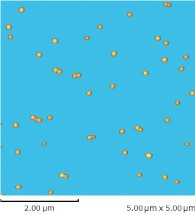Height Image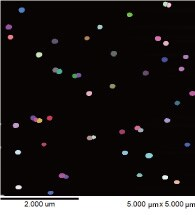Fiber Isolation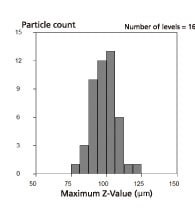Histogram of Particle Size Distribution

### Fiber Length Analysis of Cellulose Nanofibers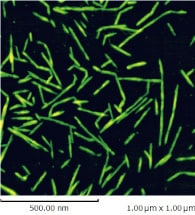Height Image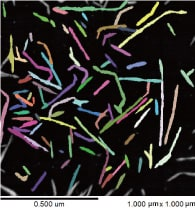Fiber Isolation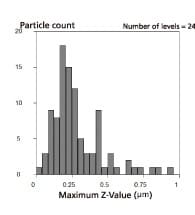Histogram of Boundary Length Distribution

This allows isolating numerous nanoparticles and nanofibers from observation data and calculating their diameter, boundary length, or other characteristic quantities. Fiber length is calculated as half the boundary length. Because it can statistically determine characteristic quantities for numerous samples, it can also be used for shape-based quality control applications.

Characteristic Quantities

1. Center of gravity X-coordinate
2. Center of gravity Y-coordinate
3. Absolute maximum diameter
4. Pattern width
5. Horizontal Feret diameter
6. Vertical Feret diameter
11. Minimum distance between centers of gravity
12. Boundary length
13. Boundary length of convex hull
14. Maximum Z-value
15. Minimum Z-value

1. Mean Z-value
2. Mean Z-value of particle boundary
3. Area excluding holes
4. Area including holes
5. Surface area
6. Volume
7. Pattern orientation
8. Angle of principal 2D moment of inertia
9. Volume ratio
10. Area ratio
11. Flatness
12. Roundness
13. Roughness
14. Thinness

Statistical Quantities

1. Mean
2. Standard deviation
3. Mean length
4. Mean area
5. Mean volume
6. Total
7. Maximum value
8. Minimum Value
9. Label number for maximum value
10. Label number for minimum value
11. Range
12. Particle count